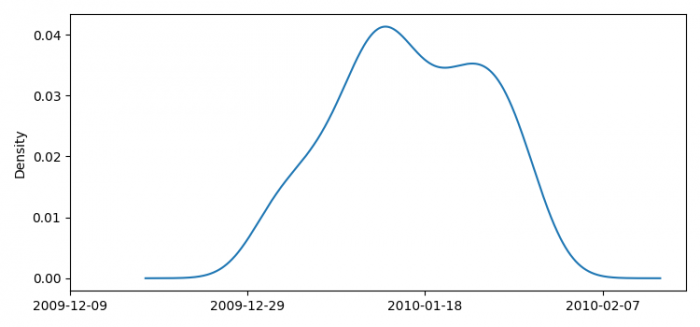# How to plot a kernel density plot of dates in Pandas using Matplotlib?

To plot a kernel density plot of dates in Pandas using Matplotlib, we can take the following steps −

• Set the figure size and adjust the padding between and around the subplots.
• Create a Pandas dataframe.
• Format the Pandas date column.
• Plot the Pandas date as kernel density estimate class by name.
• Set xtick labels using set_xticklabels() method.
• To display the figure, use show() method.

## Example

import pandas as pd
import numpy as np
import datetime
import matplotlib.pyplot as plt

plt.rcParams["figure.figsize"] = [7.50, 3.50]
plt.rcParams["figure.autolayout"] = True

dates = pd.date_range('2010-01-01', periods=31, freq='D')

df = pd.DataFrame(np.random.choice(dates, 100), columns=['dates'])
df['ordinal'] = [x.toordinal() for x in df.dates]

ax = df['ordinal'].plot(kind='kde')

x_ticks = ax.get_xticks()
ax.set_xticks(x_ticks[::2])

xlabels = [datetime.datetime.fromordinal(int(x))
.strftime('%Y-%m-%d') for x in x_ticks[::2]]
ax.set_xticklabels(xlabels)

plt.show()

## Output